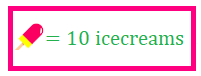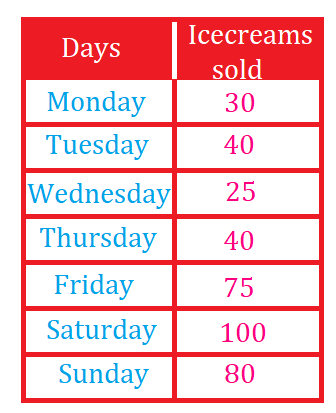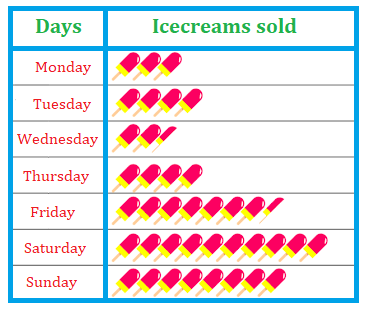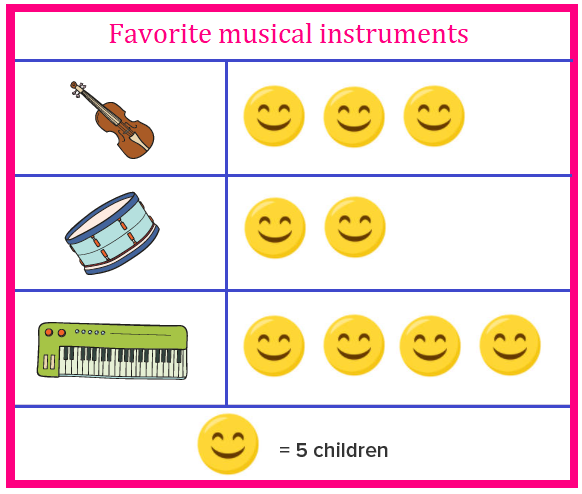# Math > Year 3

## Interpreting and Presenting data in pictograms

What is a pictogram?
A pictogram is a graph that uses pictures to represent data. It is also known as a pictograph or picto.

Key: A key tells us what the pictures in the pictogram represent.

How to present data in a pictogram?

The number of ice creams sold by an ice cream parlour is shown in the table. Represent the data in form of a pictograph taking key as:1 symbol = 10 ice creams

Represent the above number of ice creams on the pictogram.How to interpret the data represented in the pictogram?

Let’s try to answer the questions.Question 1: How many children like a guitar?

Answer: Number of symbols = 3

Each symbol represents 5 children.

Total number of children = 3 × 5

= 15

15 students like Guitar.

Question: 2 Which instrument was liked by the most number of students?

As there are most smileys (4) for Piano.

Number of students who like Piano = 4 × 5

= 20

20 Students like Piano.

//